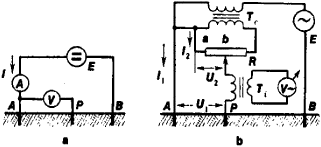# Ground Meter

The following article is from The Great Soviet Encyclopedia (1979). It might be outdated or ideologically biased.

## Ground Meter

a device for measuring earth resistance. Ground meters and measuring methods may be simple (ammeter-voltmeter methods) or complex, if used in precision measurements (compensation or bridge measurements). If the ammeter-voltmeter method is used in measurements (Figure l,a), the resistance of the ground conductor A is determined according to the formula rg = V/I, where V is theFigure 1. Diagrams of the measurement of earth resistance by the ammeter-voltmeter method (a) and the compensation method (b): (E) current source, (Tr) current transformer,(A) ground, (P) probe, (B) auxiliary ground,(R) rheostat, (Ti) insulating transformer, (I) ground current

voltage between A and the probe P in the zone of zero potential and I is the current in the grounding conductor. If the compensation method is used (Figure 1,b), the voltmeter V∽ is connected across the secondary winding of transformer Ti. A sliding contact of the rheostat R is then moved until the equality U1 = U2 is achieved. When this condition is reached, no current flows in the windings of Ti, (V∽ reads zero), and the earth resistance can be read on the scale of the rheostat, since rg = Ra.

### REFERENCES

Popov, V. S. Elektrotekhnicheskie izmereniia i pribory, 7th ed. Moscow-Leningrad, 1963.